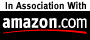# Applied Biostatistics

### Software for teaching and research

 NTSYSpc Multivariate statistics, dis/similarity (distance, correlation, association, genetic), clustering (UPGMA, single link, complete link, neighbor joining, MST, consensus), ordination (principal components, principal coordinates, multidimendional scaling, singular-value decomposition, CVA, canonical correlation, PLS, correspondence analysis), regression (multiple,multivariate, autoregression), factor analysis (rotation to orthogonal and oblique simple structure), Mantel test and correlation, transformations, graphics (2D and 3D scatterplots, dendrograms), geometric morphometrics, statistical calculator, estimate sample sizes, effect sizes. BIOMstat Basic statistics, regression (bivariate, multiple, ancova, isotonic, robust, polynomial, logistic, analysis of variance (single classification, 2-way, multiway, nested, multiple comparisons, homogeneity of variances), non-parametrics (Mann-Whitney U, Mantel, 2-way), frequency data (goodness of fit, Fisher's exact, log-linear models, logistic regression), and graphics. Based on the text Biometry by Sokal and Rohlf.

Need help? contact email.

This file was last modified on 23 September 2018.Shop at amazon.com? Convenient links: Home page:Software, Electronics